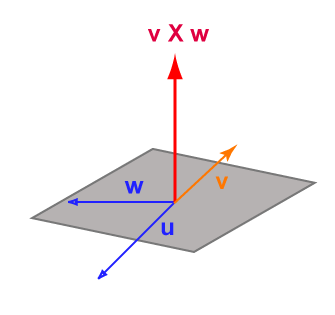By examining the diagram, or working with the formula: (ku) × v   =   k( u × v )

Fussing with math gives the result:

| (ku) × v |   =   | ku | | v | sin θ   =   | k | |u | | v | sin θ

The magnitude is | k | times the magnitude of u × v and the direction must be the same.

# Not AssociativeAnother fact is that, in general,   (u × v) × w  ≠  u × (v × w)

To see this, look at the diagram and mentally form the cross product (u × v) , then take the cross product of that with w. Then form the cross product (v × w) and take the cross product of u with that.

### QUESTION 9:

What is (u × v) in the diagram ?